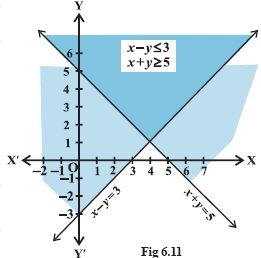Email us to get an instant 20% discount on highly effective K-12 Math & English kwizNET Programs!

#### Online Quiz (WorksheetABCD)

Questions Per Quiz = 2 4 6 8 10

### High School Mathematics - 27.6 Solutions of System of Linear Inequations in Two Variables

 Example: Solve the following linear inequalities graphically. x+y >= 5 x-y <= 3 Solution: The graph of the equation x+y = 5 is shown in the graph below.The solution of the inequality x+y <= 5 is the shaded region above the line x+y = 5 including the points on the line. On the same set of axes we draw the graph of the equation x-y = 3. The solution of the inequality x-y <= 3 is the shaded region above the line x-y = 3 including the points of the line. Directions: Draw the graph of the following inequalities.
 Q 1: Solve the following system of inequations graphically. x+y > 6, 2x-y > 0Answer: Q 2: Solve the following system of inequations graphically. 3x+y <= 60, x+3y <= 30, x > = 0, y >= 0.Answer: Q 3: Solve the following system of inequations graphically. y <= 4, x >= 1.Answer: Q 4: Solve the following system of linear inequations graphically. 2x+y-3 >= 0, x-2y+1 <= 0, y<3Answer: Q 5: Solve the following system of linear inequations graphically. y <= 4, x >= 1Answer: Q 6: Solve the following system of linear inequations graphically. 2x+y-3 >= 0, x-2y+1 < = 0, x>= 0, y >= 0Answer: Q 7: Solve the following system of inequations graphically. x+y <= 9, y > x, x >= 1.Answer: Q 8: Solve the following system of inequations graphically. 2x-y > 1, x-2y <-1Answer: Question 9: This question is available to subscribers only! Question 10: This question is available to subscribers only!

#### Subscription to kwizNET Learning System offers the following benefits:

• Unrestricted access to grade appropriate lessons, quizzes, & printable worksheets
• Instant scoring of online quizzes
• Progress tracking and award certificates to keep your student motivated
• Unlimited practice with auto-generated 'WIZ MATH' quizzes